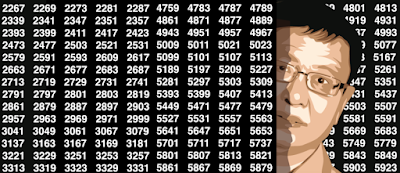## Friday, November 29, 2019

### Variance, Attractors and Behavior of Chaotic Statistical Systems

We study the properties of a typical chaotic system to derive general insights that apply to a large class of unusual statistical distributions. The purpose is to create a unified theory of these systems. These systems can be deterministic or random, yet due to their gentle chaotic nature, they exhibit the same behavior in both cases. They lead to new models with numerous applications in Fintech, cryptography, simulation and benchmarking tests of statistical hypotheses. They are also related to numeration systems. One of the highlights in this article is the discovery of a simple variance formula for an infinite sum of highly correlated random variables. We also try to find and characterize attractor distributions: these are the limiting distributions for the systems in question, just like the Gaussian attractor is the universal attractor with finite variance in the central limit theorem framework. Each of these systems is governed by a specific functional equation, typically a stochastic integral equation whose solutions are the attractors. This equation helps establish many of their properties. The material discussed here is state-of-the-art and original, yet presented in a format accessible to professionals with limited exposure to statistical science. Physicists, statisticians, data scientists and people interested in signal processing, chaos modeling, or dynamical systems will find this article particularly interesting. Connection to other similar chaotic systems is also discussed.
1. The Geometric System: Definition and Properties
• A test for independence
• Connection to the Fixed-Point Theorem
2. Geometric and Uniform Attractors
• General formula
• The geometric attractor
• Not any distribution can be an attractor
• The uniform attractor
3. Discrete X Resulting in a Gaussian-looking Attractor
• Towards a numerical solution
4. Special Cases with Continuous Distribution for X
• An almost perfect equality
• Is the log-normal distribution an attractor?
5. Connection to Binary Digits and Singular Distributions
• Numbers made up of random digits
• Singular distributions
• Connection to Infinite Random Products
6. A General Classification of Chaotic Statistical Distributions